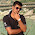Annular volume calculations

Annular volume calculation come under drilling hole volume calculation which includes calculating drill string capacity, drill string displacement and annular volume calculation.
Annular volume is the volume between tublars (drillpipe, tubing or casing) and casing and open hole.
Annular Volume (bbl)= (Dh2-Dp2)*L/ 1029.4
where, Dh=Hole diameter in inch
Dp=Drill pipe outer diameter in inch
bbl can be converted to gal by using the conversion factor:-  1 bbl= 42gal

But generally barrel and m3 are used in field as volume units as gallon is relatively smaller unit.
Several annular profiles may be present in a single hole and so the annular capacity will be different for each profile. Each annular capacity should be calculated separately.

Example:-
Annular volume across Drill pipe=(Dh2-Dp2)*L/ 1029.4
=(5.752-3.52)*1000/1029.4
=20 bbl

Annular volume across Drill collar=(Dh2-Dp2)*L/ 1029.4
=(5.752-52)*200/1029.4
=1.6 bbl

Total Annular volume=Annular Volume across Drill pipe+Annular Volume across Drill collar
=20+1.6
=21.6 bbl

1 comment:

1.